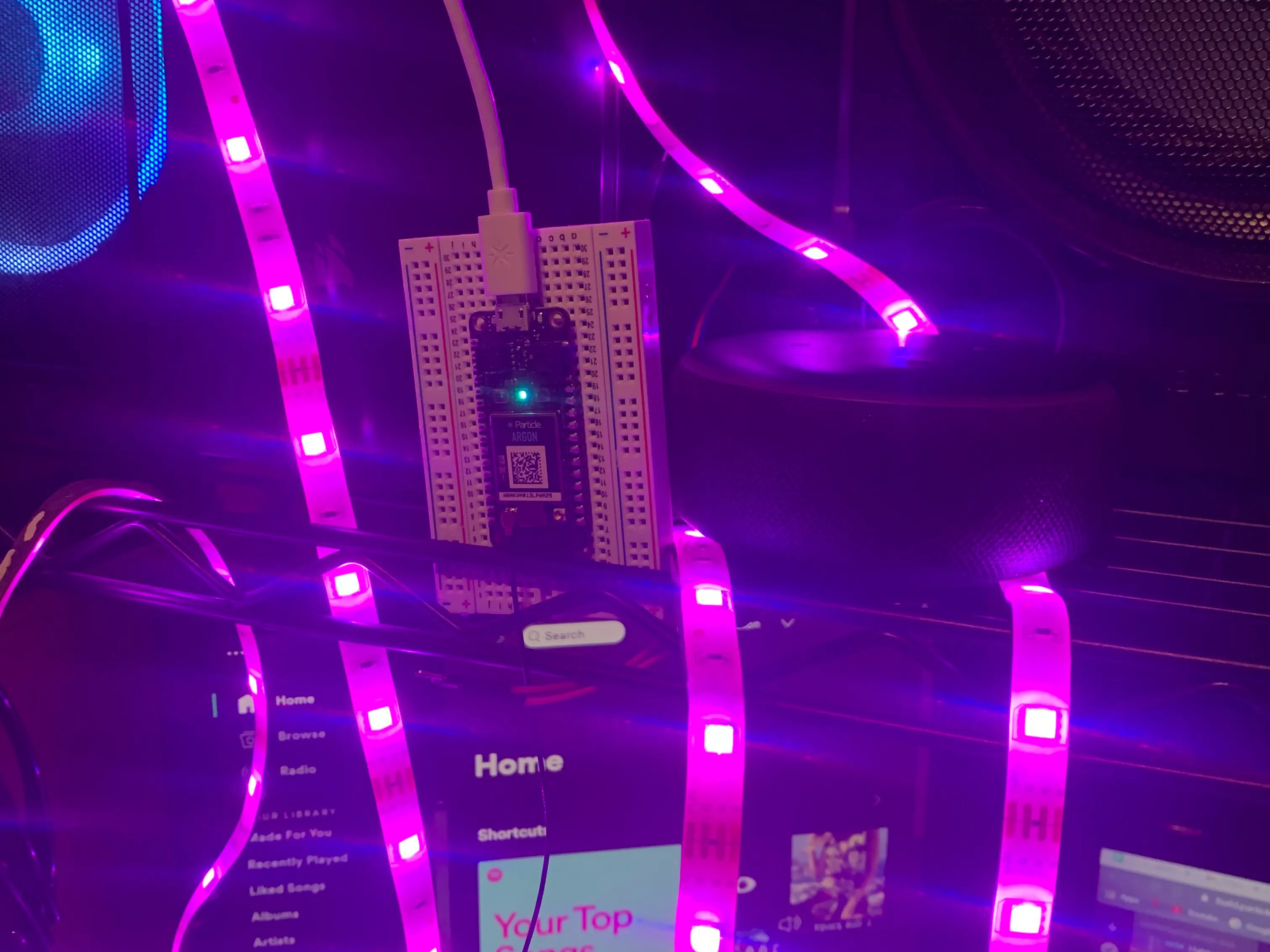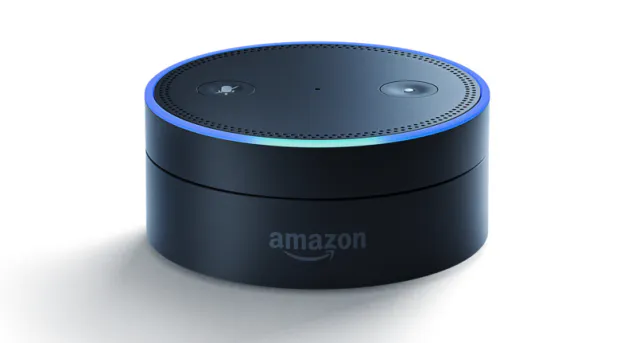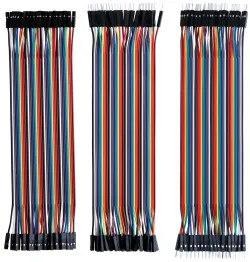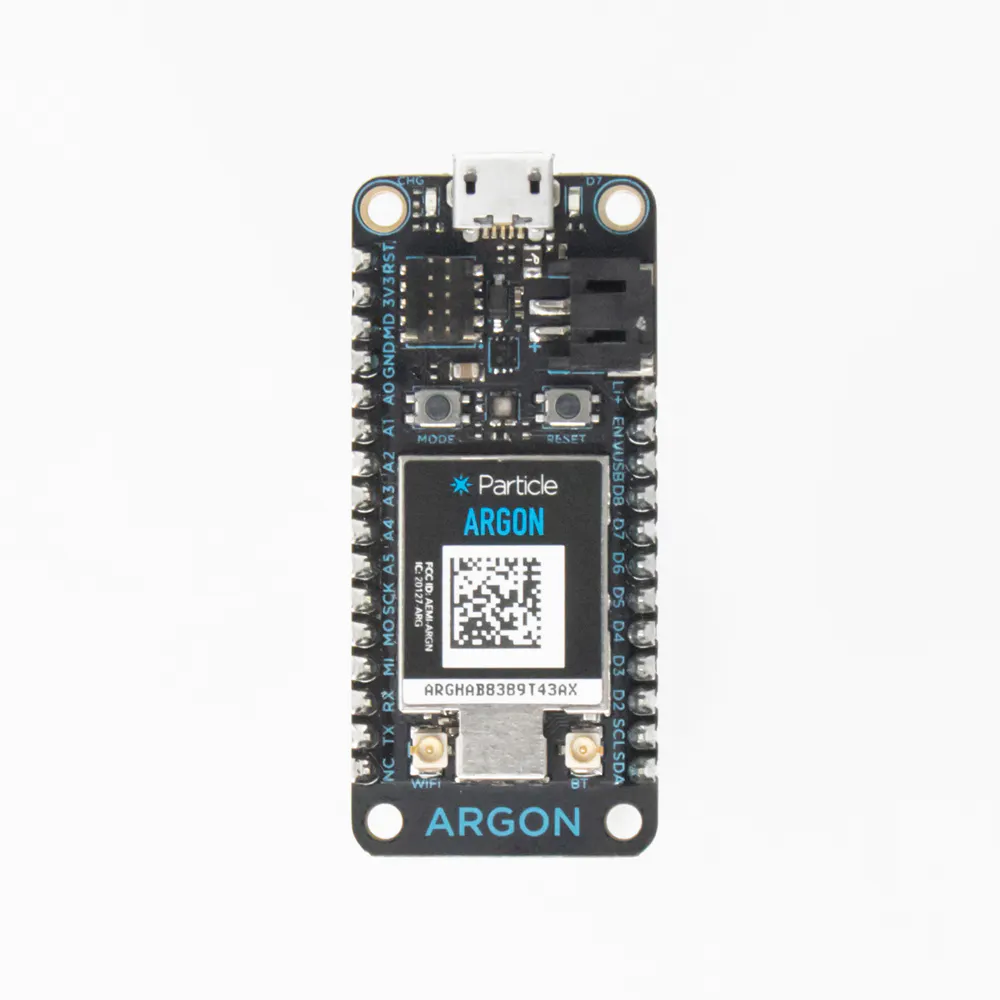Published

# Amazon Alexa Controlled LED Strip Lights

Ever wanted voice controlled LED lights? Well here you go.

IntermediateFull instructions provided228## Things used in this project

### Hardware componentsAmazon Alexa Echo Dot
×1×1ELEGOO 120pcs Multicolored Dupont Wire
×1Particle Argon
×2
 LED Strip Lights
×1

### Software apps and online servicesIFTTT Maker serviceParticle Build Web IDE## Code

### Lights

C/C++
```void trans(const char *event, const char *data){

String responsevalue = String(1);
Particle.publish("response", responsevalue, PRIVATE);
delay(2000);

if (strcmp(data, "lights_off")==0) {

analogWrite(A0, 255); //blue
analogWrite(A1, 255); //green
analogWrite(A2, 255); //red
}
else if (strcmp(data, "blue")==0) {

analogWrite(A0, 0);
analogWrite(A1, 255);
analogWrite(A2, 255);
}
else if (strcmp(data, "green")==0) {

analogWrite(A0, 255);
analogWrite(A1, 0);
analogWrite(A2, 255);
}
else if (strcmp(data, "red")==0) {

analogWrite(A0, 255);
analogWrite(A1, 255);
analogWrite(A2, 0);
}
else if (strcmp(data, "orange")==0) {

analogWrite(A0, 255);
analogWrite(A1, 127);
analogWrite(A2, 0);
}
else if (strcmp(data, "yellow")==0) {

analogWrite(A0, 255);
analogWrite(A1, 0);
analogWrite(A2, 0);
}
else if (strcmp(data, "purple")==0) {

analogWrite(A0, 0);
analogWrite(A1, 255);
analogWrite(A2, 0);
}
else if (strcmp(data, "pink")==0) {

analogWrite(A0, 127);
analogWrite(A1, 255);
analogWrite(A2, 0);
}

//hard code blinking yellow and green every 2 seconds
//analogWrite(A0,255);    // yellow
//analogWrite(A1,0);
//analogWrite(A2,0);
//delay(2000);

//analogWrite(A0,255);    // green
//analogWrite(A1,0);
//analogWrite(A2,255);
//delay(2000);

else if (strcmp(data, "party_lights")==0 || strcmp(data, "party_lights") !=0)  {

analogWrite(A0, 0); // blue
analogWrite(A1, 255);
analogWrite(A2, 255);

analogWrite(A0, 255); //off
analogWrite(A1, 255);
analogWrite(A2, 255);
delay(1500);

analogWrite(A0, 255); // green
analogWrite(A1, 0);
analogWrite(A2, 255);

analogWrite(A0, 255);
analogWrite(A1, 255); //off
analogWrite(A2, 255);
delay(1500);

analogWrite(A0, 255);
analogWrite(A1, 255); //red
analogWrite(A2, 0);

analogWrite(A0, 255);
analogWrite(A1, 255); // off
analogWrite(A2, 255);
delay(1500);

analogWrite(A0, 0);
analogWrite(A1, 255); // aqua
analogWrite(A2, 127);

analogWrite(A0, 255);
analogWrite(A1, 255); // off
analogWrite(A2, 255);
delay(1500);

analogWrite(A0, 0);
analogWrite(A1, 255);  // purple
analogWrite(A2, 0);

analogWrite(A0, 255); // off
analogWrite(A1, 255);
analogWrite(A2, 255);
delay(1500);

analogWrite(A0, 255); //orange
analogWrite(A1, 127);
analogWrite(A2, 0);

analogWrite(A0, 255); // off
analogWrite(A1, 255);
analogWrite(A2, 255);
delay(1500);

analogWrite(A0, 255);
analogWrite(A1, 0); // yellow
analogWrite(A2, 0);

analogWrite(A0, 255);
analogWrite(A1, 255); // off
analogWrite(A2, 255);
delay(1500);

analogWrite(A0, 0);
analogWrite(A1, 255); // light blue
analogWrite(A2, 127);

analogWrite(A0, 255);
analogWrite(A1, 255); // off
analogWrite(A2, 255);
delay(1500);

analogWrite(A0, 0);
analogWrite(A1, 0); // white
analogWrite(A2, 0);

analogWrite(A0, 255);
analogWrite(A1, 255); // off
analogWrite(A2, 255);
}
else {}

delay(1000);
}

void setup() {

pinMode(A0, OUTPUT); //blue
pinMode(A1, OUTPUT); //green
pinMode(A2, OUTPUT); //red

}
```

### Communication

C/C++
```void lights(const char *event, const char *data){

Particle.publish("transmission", data, PRIVATE);
delay(1000);

}

void responce(const char *event, const char *data){

if (strcmp(data, "1")==0) {

digitalWrite(D7, HIGH);
delay(500);
digitalWrite(D7, LOW);
delay(500);

}

else {}

}

void setup() {

pinMode(D7, OUTPUT);

}

void loop() {

Particle.subscribe("party_lights", lights, MY_DEVICES);
delay(1000);
Particle.subscribe("blue", lights, MY_DEVICES);
delay(1000);
Particle.subscribe("green", lights, MY_DEVICES);
delay(1000);
Particle.subscribe("red", lights, MY_DEVICES);
delay(1000);
Particle.subscribe("orange", lights, MY_DEVICES);
delay(1000);
Particle.subscribe("yellow", lights, MY_DEVICES);
delay(1000);
Particle.subscribe("pink", lights, MY_DEVICES);
delay(1000);
Particle.subscribe("purple", lights, MY_DEVICES);
delay(1000);
Particle.subscribe("light blue", lights, MY_DEVICES);
delay(1000);
Particle.subscribe("response", responce, MY_DEVICES);
delay(1000);
}
```